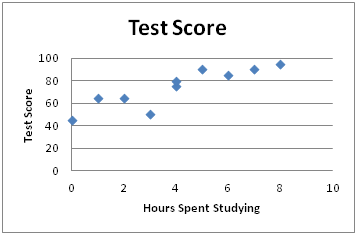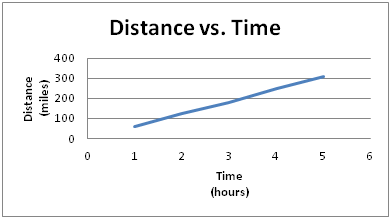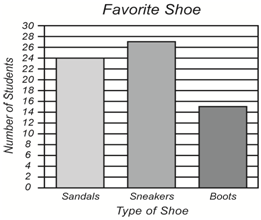Discrete Paired Data

My Definition

Key Characteristics

The following are true of discrete paired data:

• It features pairings of quantitative or numerical data.

• It can be a sequence which is countably infinite.

• The data set is represented graphically with unconnected data points.

• Discrete data is counted.

• It is not continuous.

Example

Discrete Paired Data Card Sort Activity

The scatterplot below represents discrete paired data.Non-example

Paired measures, such as time and distance, represent continuous data.

The graph below represents continuous data.While the bar graph below represents discrete data, it does NOT represent discrete paired data.TEKS: 5(9)(B)